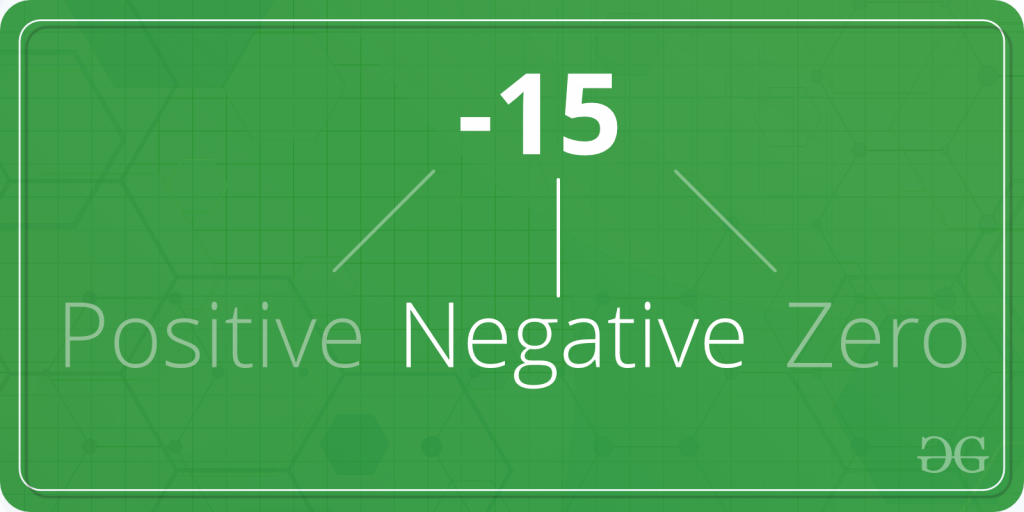# C program to Check Whether a Number is Positive or Negative or Zero

Given a number A. The task is to check whether A is positive, negative or zero.Examples:

```Input: A = 2
Output: 2 is positive

Input: A = -554
Output: -554 is negative
```

## Recommended: Please try your approach on {IDE} first, before moving on to the solution.

In the below program, to find whether A is positive, negative or zero; first the number is taken as input from the user using scanf in, and then A is checked for positive usingstatement and,andoperators.

Below is the C program to find whether a number is positive, negative or zero.

 `#include ` ` `  `int` `main() ` `{ ` `    ``int` `A; ` ` `  `    ``printf``(``"Enter the number A: "``); ` `    ``scanf``(``"%d"``, &A); ` ` `  `    ``if` `(A > 0) ` `        ``printf``(``"%d is positive."``, A); ` `    ``else` `if` `(A < 0) ` `        ``printf``(``"%d is negative."``, A); ` `    ``else` `if` `(A == 0) ` `        ``printf``(``"%d is zero."``, A); ` ` `  `    ``return` `0; ` `} `

Output:

```Enter the number A =: -54
-54 is negative.
```

My Personal Notes arrow_drop_upTechnical Content Engineer at GeeksForGeeks

If you like GeeksforGeeks and would like to contribute, you can also write an article using contribute.geeksforgeeks.org or mail your article to contribute@geeksforgeeks.org. See your article appearing on the GeeksforGeeks main page and help other Geeks.

Please Improve this article if you find anything incorrect by clicking on the "Improve Article" button below.